## Fluids

Tagged:

•michutorres
Subscriber

Hi all,

My work consists on a adhesive melting simulation in a deposit. The adhesive should start solid and becoming fluid as it gets warmer because a heat flux inside the deposit of aluminium.

When a run calculation in Fluent, I am getting an error that says 'Floating point exception'. I am going to explain all the set up.

•YasserSelima
Subscriber
Time step size is too large. make it 0.001 ... and maximum number of iterations, at least 20n
•michutorres
Subscriber
Ok I will try this.nIn reality, adhesive takes 30 minutes to get totatlly melted, so that means 1800 seconds. If I put 0.001 time step size, I would need 1.800.000 Number of steps. That would be ok?n
•YasserSelima
Subscriber
Try to get convergence first, then use adaptive time step and let fluent decide the optimum sizen
•michutorres
Subscriber
What do you mean with 'try to get convergence first'? How can I ckeck this?n
•YasserSelima
Subscriber
I mean make sure solution do converge first. Try few time steps ... make sure the residuals decrease every time step until they reach constant value or go below the set criteria. nThen think how to optimise the solutionn
•michutorres
Subscriber
Solution didn't converge. Can you please recommend me which models I should enable for my case? I enabled Energy and Solidifcation & Melting.nWhat about viscous (laminar)?nI am not understanding why it doesn't converge.n
•YasserSelima
Subscriber
If you have laminar flow, use laminar.nMake the density as a function of temperature for your material. this is important to model natural convection in a liquid.n
•michutorres
Subscriber
Ok I will change the viscous model from k-epsilon to laminar.nFor the density definition I used Piecewise-linear and I introduced 2 points depending the temperature (K) (910 kg/m3 at 313 K and 790 kg/m3 at 398 K).nI don't have any natural convection. All faces are adiabatic. The only one boundary condition that I have in my model is a heat flux in a wall inside the deposit.nWhat do you think?n
•YasserSelima
Subscriber
For any fluid, if the density changes with the temperature and there is a room for circulation, natural convection will occur n
•michutorres
Subscriber
Yes I know. I thought you were talking about a convection between the air and the adhesive. nDo I have to insert the convection coefficient h of the contact adhesive-deposit? Or is the program who calculates it once the adhesive starts to get melted? n
•YasserSelima
Subscriber
no you don't have to, but you need to insert the thermal properties in the material paneln
•michutorres
Subscriber
ok thank you.nI got the density as a function of temperature: Density=3'9286 T^2-57'929 T +984nDo I have to create a Named Expression or UDF? Or can I write this formula directly in the material properties panel selecting piecewise linear or polynomial or bussineseq? n
•YasserSelima
Subscriber
In the material panel , polynomial ... Bussineseq will not work in your case, you have large temperature differencen
•michutorres
Subscriber
Ok I introduced the density as a polynomial function. For the viscosity and heat capacity I did the same but the plot was not available. n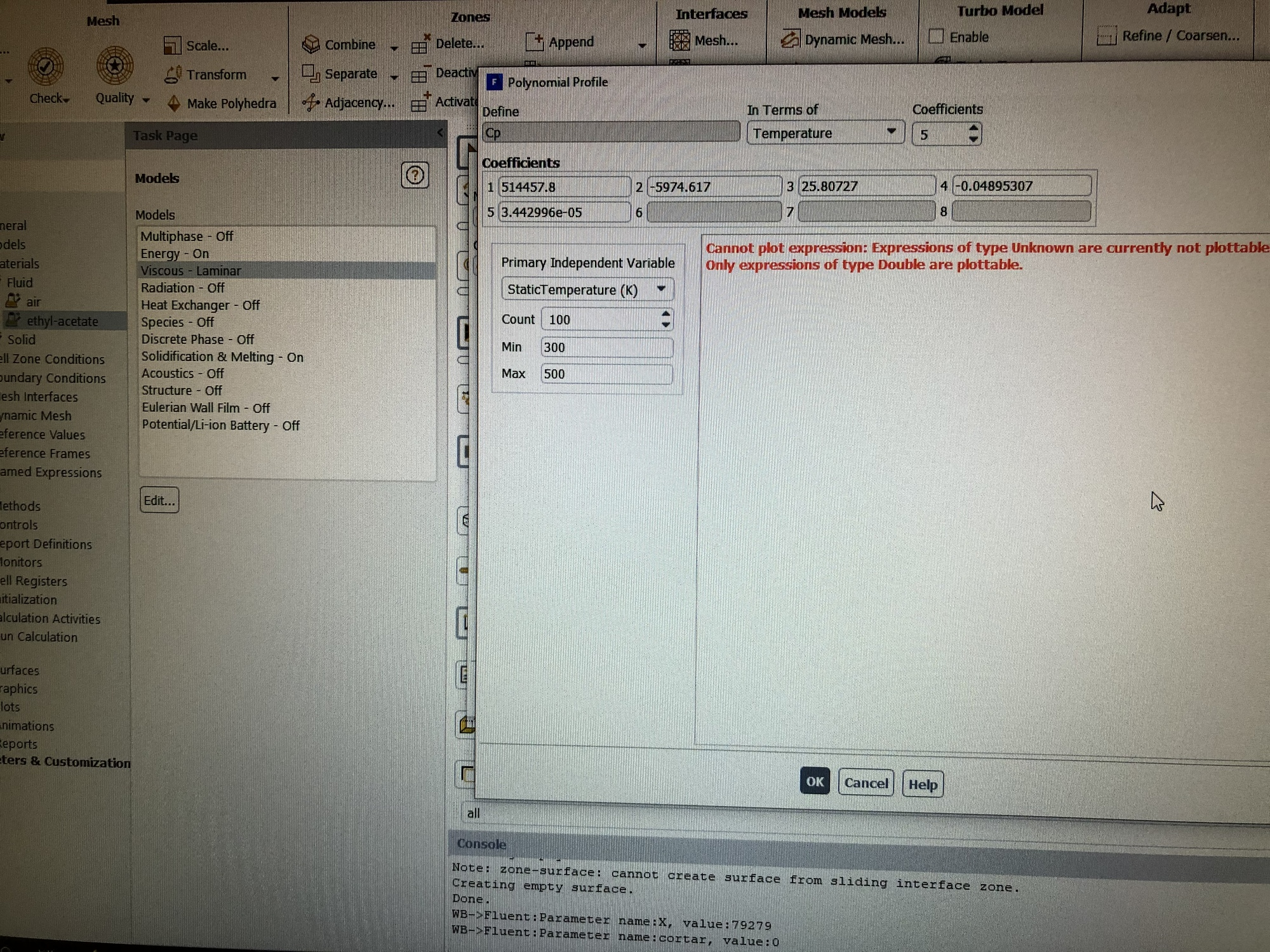Is this a problem?nby the way, I changed to laminar and introduced the density as a function and the error of floating point exception continues appearing...nwhat can I do?n
•YasserSelima
Subscriber
Not a problem. nThe floating point exception can occur because of many many reasons. Defining the density as a function of temperature or not, might not be one of these reason. It is a matter of defining the physics of the problem.nDo transient simulation and decrease the time step to a very small value and increase the number of iterations to more than 100. Run few time steps with this conditions. If you get floating point excption, you will need to refine the mesh and try againn
•michutorres
Subscriber
Ok thank you.ni will try the following:nnumber of time steps 20ntime step size: 0.00001nmax iterations 150ni will run this few times.nthat would be correct?.by the way, in Methods I selected Scheme=PISO, pressure= PRESTO, Momentum = Third Order Muscl and Energy =Third Order Muscl. nCould it be this the problem?nThank you
•YasserSelima
Subscriber
increase the number of iterations.nUse first order when applicable. This helps getting convergencen
•michutorres
Subscriber
Hi,nI changed the Method using First Orders and I set up the Run Calculation like this:n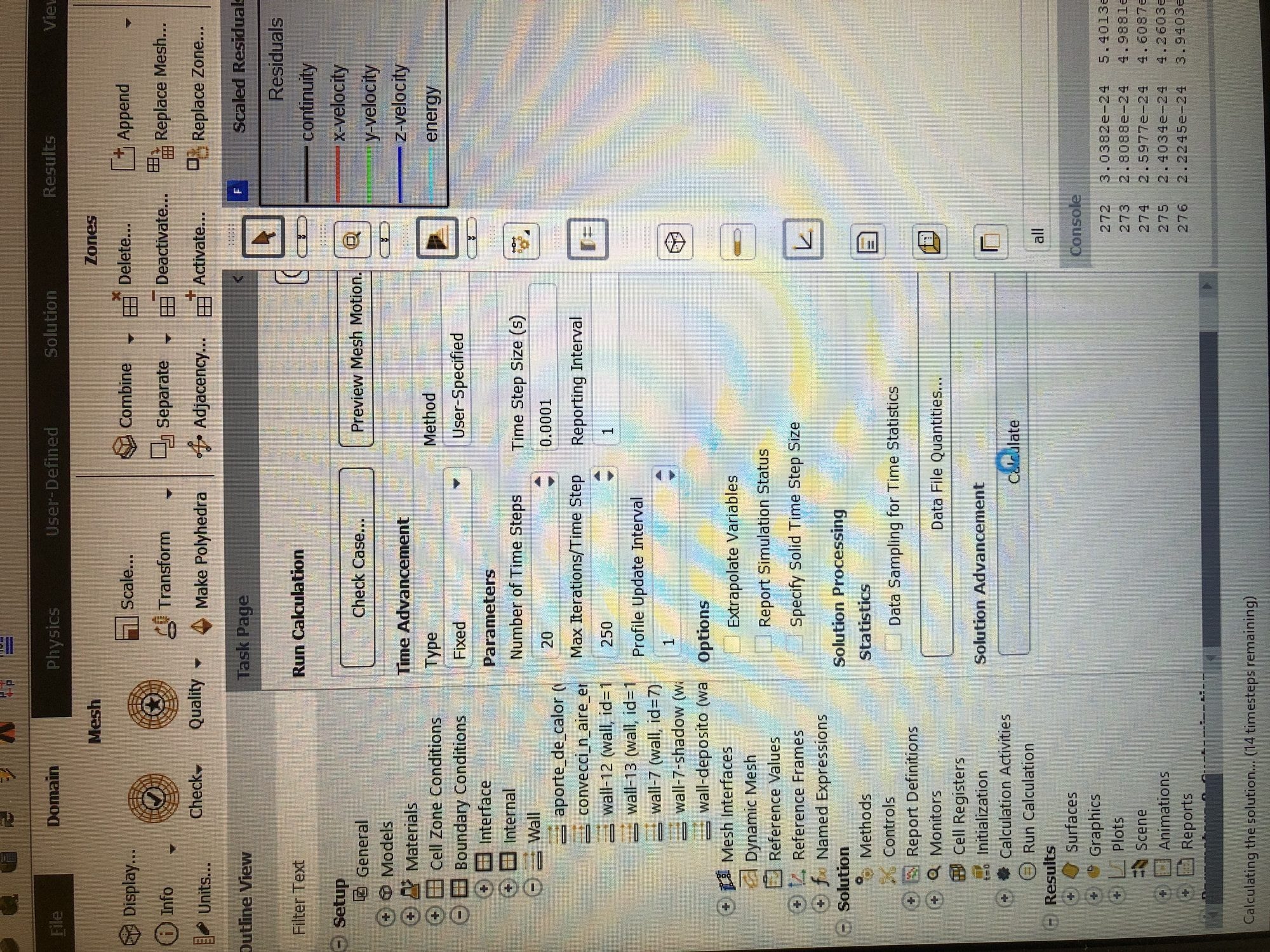Now the Scaled Residuals looks better but everytime that it says ‘solution is converged’ I can see a peak in the plot. Is it normal? n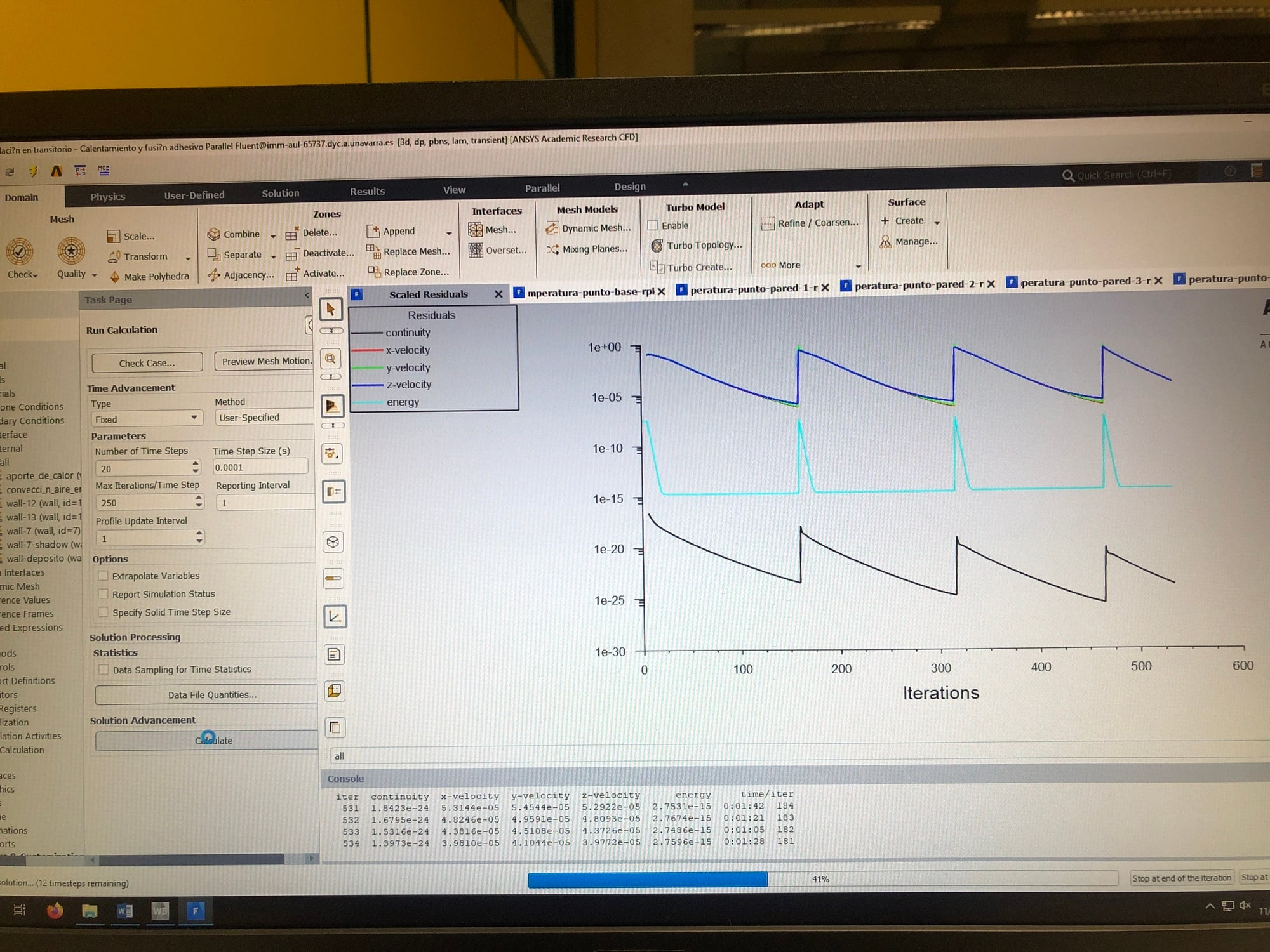nIf this is normal, which is my next step? You told me to use adaptive time step and let the fluent decide the optimum size. How can I do this?nmany thanksn
•Rob
Ansys Employee
That's normal, the residual will spike for each time step and then converge. As you're changing the material density where does the extra volume go in your model? n
•michutorres
Subscriber
Hi Rob,nI changed the Run Calculation parameters as I want to simulate 30 minutes (1800 seconds):nNumber of Time Steps: 1800000nTime Step Size (s): 0.001nMax Iterations/Time Step: 20nBut the residuals increase:n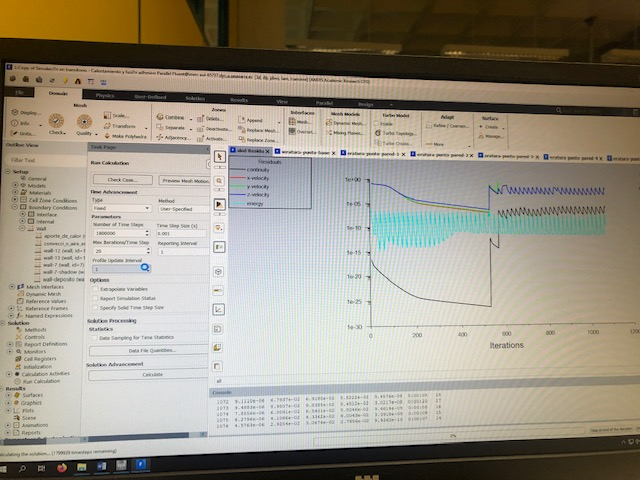So, I don't know which parameters should I set in order to simulate 1800 seconds.nnConcerning the material density change, I didn't think about the extra volumen that will appear. How can I consider this extra volume in Ansys Fluent?nThank youn
•Rob
Ansys Employee
If you're not converging the time steps you most likely need to decrease the time step, failure to converge the step will result in the data becoming increasingly inaccurate. nRe the extra volume, you could use VOF and allow the liquid to expand into an open gas space, or for ease you can also just add a flow boundary and monitor how much mass you lose. Remember to turn on gravity! n
•michutorres
Subscriber
1) As far as I know, I need to 'play' with time step size and iteration to reach convergence. With time step=0.0001s and 250 iterations, solution converges. But if I increase time step to 0.001s keeping 250 iterations, residuals become crazy. That means that if I want to decrease time step I should increase iterations? With these values the simulation will take too much time (I need to simulate 1800 seconds).n2) What do you mean with a flow boundary? Can you give me an example? And how do I know how much mass will be lost?n3) I set a heat flux in a boundary condition (wall). Should I enable the shell conductivity?n
•michutorres
Subscriber
Hi,nHow can I add a flow boundary and monitor how much mass I lose in order to consider the extra volume?nThank youn
•YasserSelima
Subscriber
He meant by making the top surface outlet pressure, you can monitor the amount of mass going out.nTo use Adaptive time step, in your posted photo of the calculation tab, Do you see the word Fixed? change it to adaptiven
•michutorres
Subscriber
The amount of mass going out is something that I have to calculate and introduce in the pressure outlet boundary condition?n
•YasserSelima
Subscriber
No, you can monitor the mass flow on the boundaryn
•michutorres
Subscriber
What do you mean with monitor the mass flow?n
•YasserSelima
Subscriber
report definition - area integral - mass flux .. and select the surface .. check plotn
•michutorres
Subscriber
creating the pressure outlet boundary condition and then monitoring the mass flow is mandatory? nor I can leave it as it is without considering the extra volumen
•YasserSelima
Subscriber
You can leave it. I am just explaining what was suggested by Rob.n
•Rob
Ansys Employee
You want to converge each time step in 10-15 iterations, otherwise you risk needing many more iterations to reach the end time for your calculation. There is no need to consider fluid expansion, but if the density varies with temperature the extra volume has to go somewhere. n
•michutorres
Subscriber
Hi,nlook at my residuals. Do you think they are too high to obtain a good results?n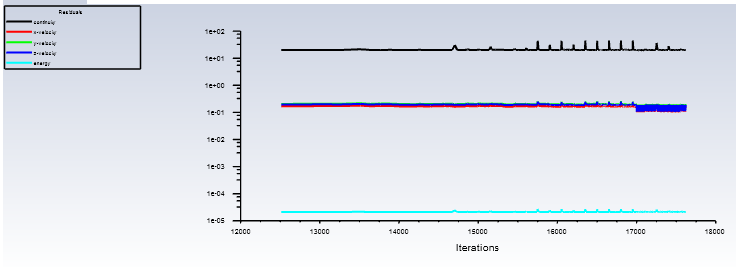By the way, I ran the simulation with adaptive method and it goes really slow... In 10 hours of real life, the simulation is now at 11 seconds (it has to reach 1800 seconds). How can I improve this (make it quicker)?n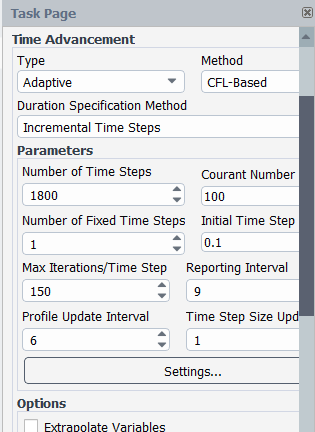n
•Rob
Ansys Employee
That residual is way too high, you're aiming for 0.001 or so. Roughly how long does it take the flow to cross one cell? n
•michutorres
Subscriber
Hi,nI got some results on my project. Remeber that my project consists on the heating and melting of adhesive because of a heat flux inside the deposit.n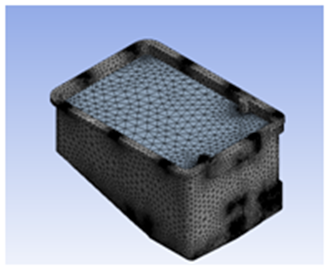nThis graph corresponds to the evolution of temperature of a point of one deposit wall and it is ok because in real life it takes 600 seconds to reach 150 ºC = 423K. At this temperature we turn off and turn on the heat flux so that it acts like a thermostate.n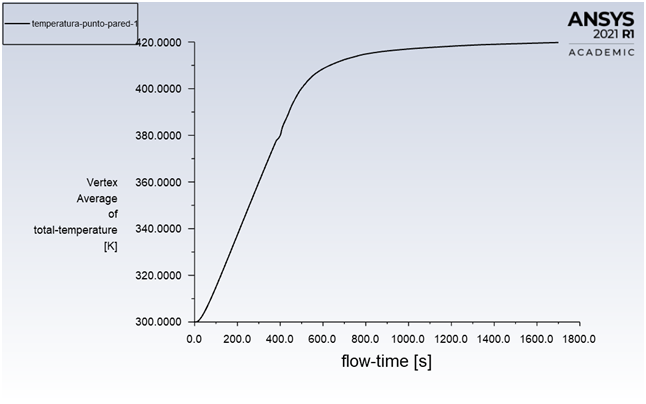The problem that I am having is that the temperature of a point in the adhesive body don't reach 423K as it is supposed. In real life, the melting of the adhesive takes less than 1800 seconds (30 minutes). What can I change in Fluent so that I get valid results for the adhesive temperature graph?n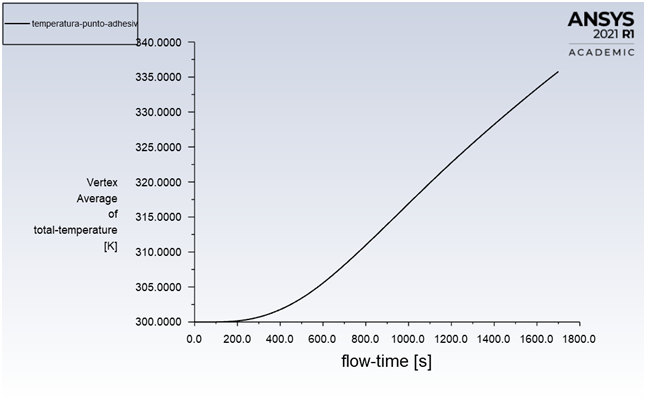Thank youn
•Rob
Ansys Employee
Where is the point? Remember as the adhesive starts to melt that convection currents will start up which may increase heat transfer within the fluid zone. n
•michutorres
Subscriber
The point it is in the middle of the adhesive part.nThe adhesive melting temperature is 105?C (378K). We can see in the previous graphics that the adhesive temperature goes from 26 ?C (300K) to 67?C (340K) in 30 minutes. Something is wrong here.n